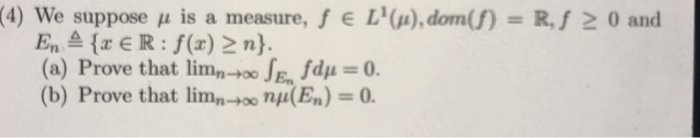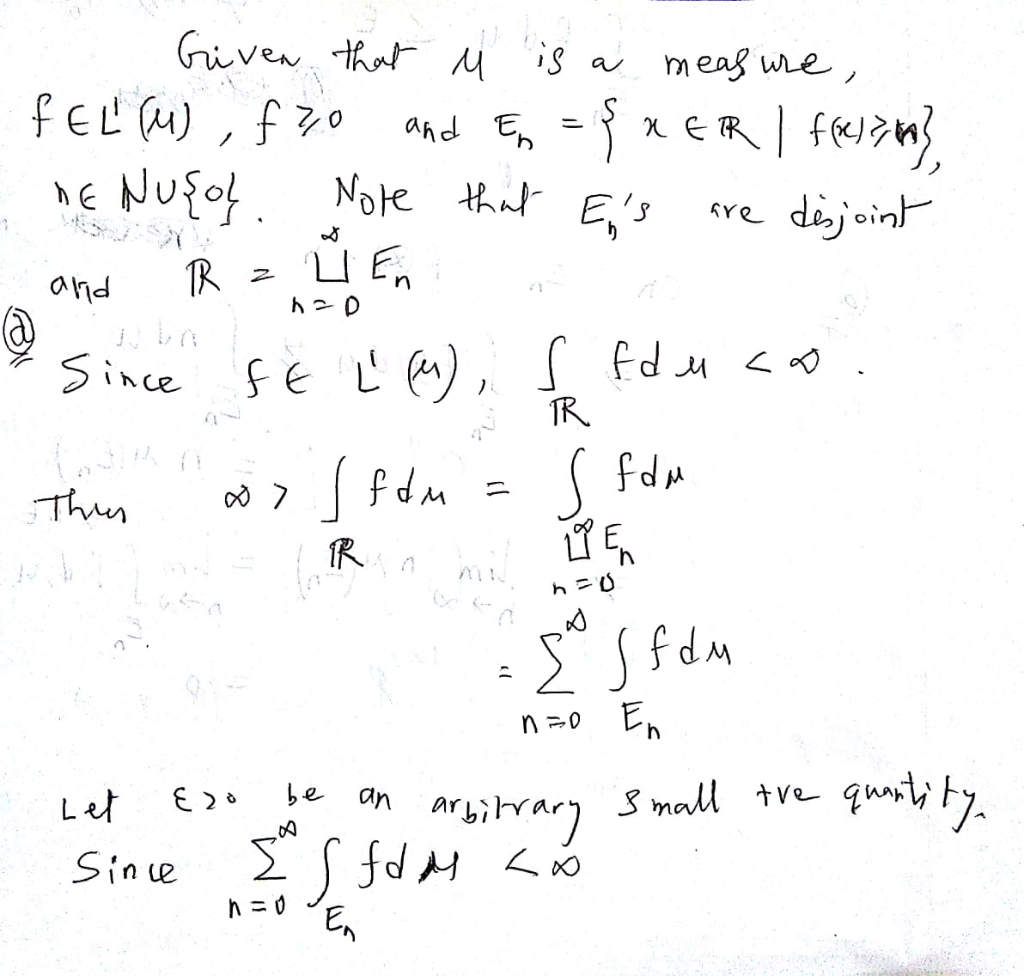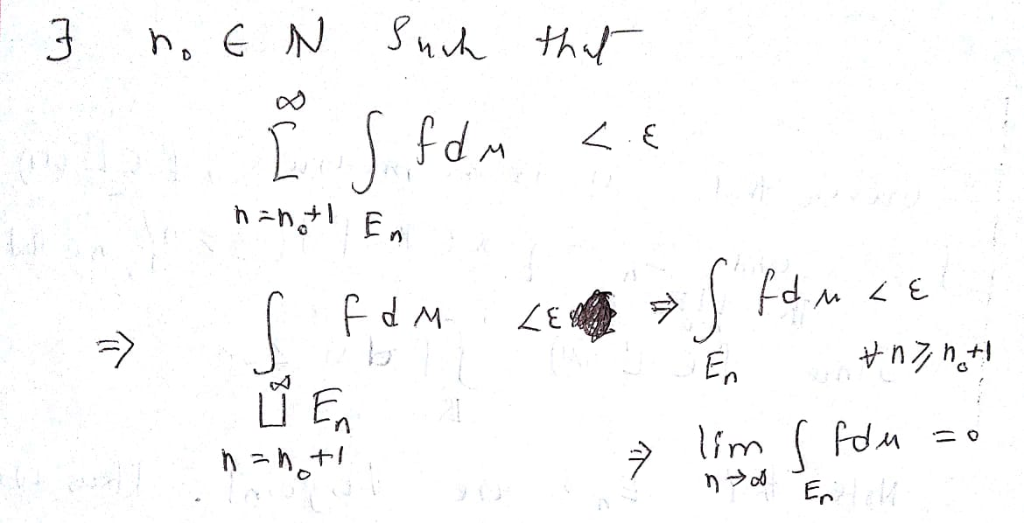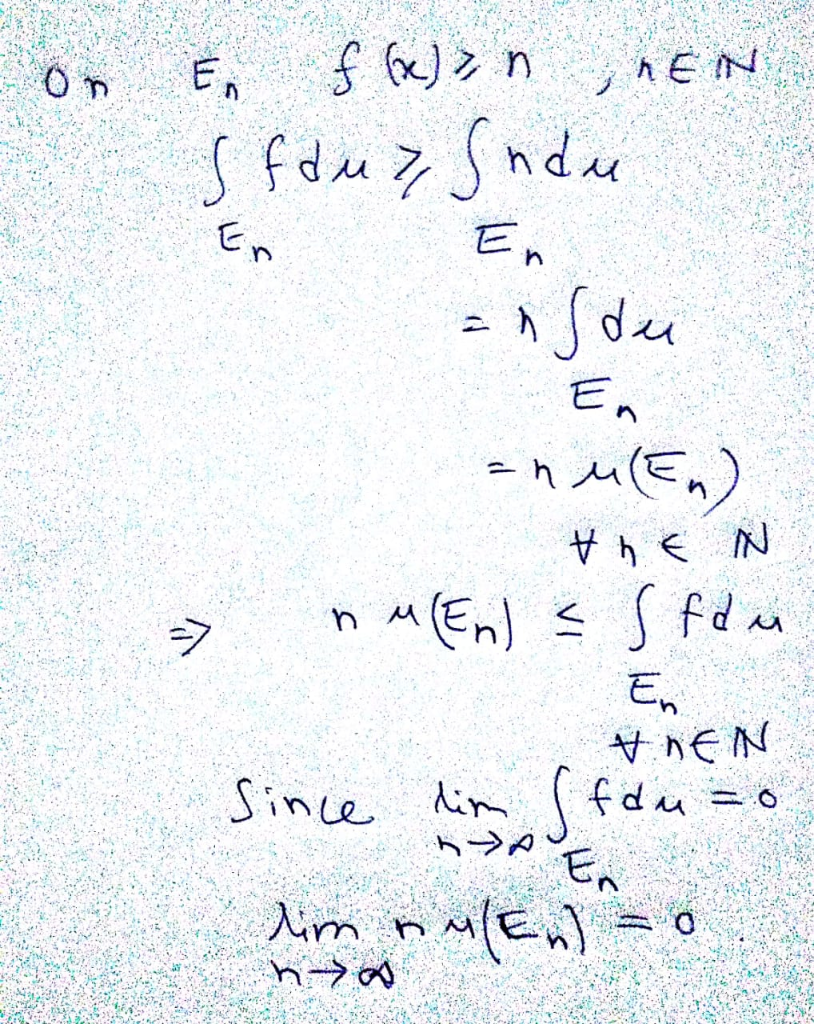Homework Help Question & Answers

# (4) w e suppose j is a measure, f E L1(μ),dom(f)-R, f 0 and EnaER: f(x) 2 n). a) Prove that limn-...(4) w e suppose j is a measure, f E L1(μ),dom(f)-R, f 0 and EnaER: f(x) 2 n). a) Prove that limn-100 JE, fdy -0 (b) Prove that limn-0o n(En)-0

#### Homework Answers

ReportAnswer #1Add Homework Help Answer
##### Add Answer of: (4) w e suppose j is a measure, f E L1(μ),dom(f)-R, f 0 and EnaER: f(x) 2 n). a) Prove that limn-...
Your Answer: Your Name: What's your source?
Not the answer you're looking for? Ask your own homework help question. Our experts will answer your question WITHIN MINUTES for Free.
More Homework Help Questions Additional questions in this topic.

• #### Find the measure of angle DBC if the measure of angle ABD is 36 degrees and the measure of angle ABC is 74 degrees

Need Online Homework Help?

Get FREE EXPERT Answers
WITHIN MINUTES
Related Questions